Study the Properties of Water Mist Droplet by Using FDS | OMICS International
Journal of Civil & Environmental Engineering

# Study the Properties of Water Mist Droplet by Using FDS

 Mohamed Fayek AbdRabbo, Ayoub Mostafa Ayoub, Mohamed Aly Ibrahim, and Abdelsalam M. Sharaf eldin* Benha University, El-Shaheed Farid Nada, Banha, Al Qalyubia Governorate 1351, Egypt *Corresponding Author : Abdelsalam M. Sharaf eldin Benha University El-Shaheed Farid Nada Banha, Al Qalyubia Governorate 13511, Egypt Tel: +20 13 3231011 E-mail: [email protected] Received: April 06, 2016; Accepted: April 20, 2016; Published: April 22, 2016 Citation: AbdRabbo MF, Ayoub AM, Ibrahim MA, Sharaf eldin AM (2016) Study the Properties of Water Mist Droplet by Using FDS. J Civil Environ Eng 6:224. doi:10.4172/2165-784X.1000224 Copyright: © 2016 AbdRabbo MF, et al. This is an open-access article distributed under the terms of the Creative Commons Attribution License, which permits unrestricted use, distribution, and reproduction in any medium, provided the original author and source are credited.

Visit for more related articles at Journal of Civil & Environmental Engineering

#### Abstract

Fire suppression systems are an extremely important part of every building that ensures the safety of the occupants and limits damage due to fire. Full scale model is set up for the purpose of predicting the geometry of fire spread and water mist particles distribution and movement. The results are based on full scale model simulation for several properties of water mist droplet. The water mist droplet speed, mist flux, droplet size and droplet distribution are discussed. It can be concluded that The water mist droplet movement, particle size and droplet distribution play a vital role to suppress fire.The present study is carried out using Fire dynamic simulator (FDS) to perform the model of room fire scenario require to investigate and compute particle distribution, droplet velocity, mist flux and droplet size.

 Keywords Droplet; FDS; Particle; Water mist Introduction The effects of water mist characteristics on fire suppression for room fires are very important especially water mist droplet. The water mist droplet characteristics of the atomizer, such as dispersion, droplet movement or droplet dynamic, droplet distribution and mean particle diameter will discuss in this chapter. A numerical simulation for a model room with a single water mist nozzle will conduct in order to study the droplet characteristics. The water mist systems, often the immediate interest is in the size of the water droplets being produced as this factor greatly effects how the spray will interact with the fire spread and which of the extinguishment mechanisms will play significant roles. Many researchers have studied the fire suppression by using water mist in compartment. Simo Hostikka et al.  presented enhancements by using FDS to describe and validate the dynamic of water spray, air entrainment and radiation attenuation. Three types of nozzles are presented in this system (LN-2) with three pressures A, B and C from Marioff Company. Flow rate and particle size distributions are determined. Wighus et al.  investigated in response to the need for a fire suppression system for diesel turbines on board offshore drilling platforms. The experiments tests carried out by Wighus et al. confirmed that water mist could be used to successfully suppress fires in turbines. Water mist provides a good fire suppression solution for this type of scenario as it can be continually injected (unlike gas systems which have limited operating time), and provide less thermal shock to the turbine blades than standard sprinklers. The mechanisms of water mist involved in suppression include cooling and steam displacement and dilution of oxygen. Chuka et al.  studied the effects of the particle size and injection orientation by using water mist extinction of liquid pool fires. The base injection of particles develops the extinction effectiveness by as much as two times compared with the top injection, and small droplets were more effective in every orientation. Simpson et al.  studied the effects and efficiency of water mist used within telecommunication and electrical cabinets through experimental testes. In each test case the fire within a 2.44 m high, by 0.61 m wide and 0.46 m length electrical cabinet were all extinguished within less than two seconds using less than one liter of water of particular relevance in this set of experiments is that while the low current trips on the circuit boards activated, the current was so low that electric shock was not considered a perceivable danger. In addition to these extinguishment characteristics, after a short period of drying out, all of the electrical systems could be switched back on and continued to function. Petra Anderson et al.  studied the effectiveness of water mist injection at low level into a 1/3 scale ISO room with an open door. It simulated different fire positions, nozzles positions, water mist densities and droplet sizes in. This research also reviewed how obstacles affected the water mist particles. The results of these research indicated that a water mist densities of between 100 - 200 g/m3 were needed for suppression of a diffusion flame using fine water mist (fine particles). The droplet diameter decreased with Lower values of water mist densities. Calculations indicated that for the water particles to follow the airflow around an obstacle the particles needed to be in the region of 1- 20 μm. Based on these findings they suggest the ideal water droplet size for a low level total flooding system is between 10 – 20 μm. They also noted, however, that momentum needed to be given to the spray for it to reach all areas of the room, as other distribution modes were very slow. This research provides the most information on water mist interaction in relation to the experiments covered in this study. Evans et al.  studied the injection of water mist into a methane flame through nozzles that could be located in different positions. The experiments showed and described that the most effective direction for the water mist to be injected was vertically up, in line with the flame. The required mass rate water to mass rate of methane in this test was approximately four for extinguishment. In comparison horizontal injection resulted in an extinguishment ratio of 9.5 and opposed flow injection resulted in an extinguishment ratio of 20. This indicated that the flow patterns in relation to the reactants were very important to the success of extinguishing the fire. Hua et al.  used a numerical model to investigate the influence of nozzle characteristics on fire suppression. The mono disperse spray was treated using a Lagrangian’s approach. They concluded that a water spray with a solid cone pattern and a finer water droplet is more efficient in suppressing fires than a hollow cone pattern and a coarser water size. This research explores the possibility of using the computational fluid dynamics program Fire Dynamics Simulator (FDS) as a computational design. FDS has been used for various applications including modeling smoke flow in multi-floors buildings, tunnel fires and different ventilation conditions. FDS Model The FDS model room dimension is 2.6 m x 2.6 m x 2.4 m (length x width x height) and a door opening is located at the front of the compartment. The fire is modeled with a cubic heat source, the fire source has dimension of 0.2 m x 0.2 m x 0.6 m (length x width x height) (Figure 1). A water mist nozzle is used where is locate at the 2.3 m from the base of the center of room. The fuel is used in the simulation is methane. The specifications of nozzle will use in chapter four are {k-factor = 0.433, spray angle = 12 degree, droplet diameter = 79 μm} . The fire takes places at the end of compartment which ignite fire in couch .The door in all cases are taken to be fully opened, as given in Figure 1. Grid Resolution A 0.05 m grid is specified for the modeling normal room, with a total of 135000cells. This simulation took around 48 hours each to run. The selection of mesh size get from FDS user guide , it states to use a D*/dx ratio between 4 and 16 to calculate the appropriate mesh size. In that ratio, dx is the nominal size of a mesh cell, and D* is a diameter of fire defined in the equation (1):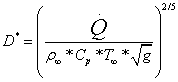(1) Where .Q is heat release rate ( HRR), ρ ∞ is the air density ( 1.204 kg/m3), Cp is the air specific heat (1.005 kJ/kg.K), T∞ is the ambient temperature(293 K), and g is the acceleration due to gravity(9.81 m/s2). The finest mesh size we would have to use is with a D*/dx ratio of 16. Governing Equations of Model This part discusses the basic of conservation equations for energy, mass, and momentum for a Newtonian fluid .The description of the equations, the notation, and the different approximations employed by Anderson et al. . A six differential equations for six unknowns, all functions of three spatial dimensions and time: the density (ρ), the three velocity components U = [u; v; w], the temperature (T), and the pressure (p). The continuity equation can be simplified as the following relation: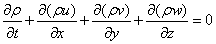(2) When the flow is at steady -state, ρ does not change with respect time. The equation is reduced to: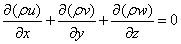(3) The momentum equations with the components of velocity are typically named u, v , w. while the three equations are :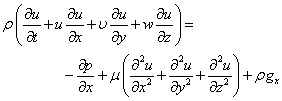(4)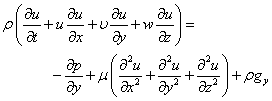(5)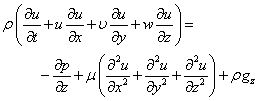(6) Note that gravity has been accounted for as a body force, and gx,gy,gz values will be depend on the orientation of gravity with respect to chosen set of coordinates. The energy equation in a multi-component reacting system, there are several mechanisms that contribute to the total heat flux, the most common known as conduction, convection and radiation. Mainly two additional effects are encountered in the literature; these are the effect of mechanical work done on the system due to buoyancy and the socalled Dufor effect. The latter describes the heat flux in a system due to concentration gradients and in general, this term can be neglected due to the low velocities involved in a fire the mechanical work term can be ignored as well. The energy equation can be written in different ways depending on which quantity is used as the dependent variable. Using the total enthalpy, htotal = cpT+ΣYα Hr where Hr is the component heat of reaction , as dependent variable , the conservation of energy equation becomes: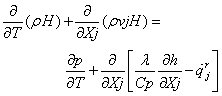(7) The program goes deeply in calculating all species quantities using complicated equation which will be very easy to be solved now a days by PC’s other than manual old method. The program relations and equations can be found on site in a PDF file  for more details. Water Mist Droplet Models The technicality of energy transfer between the water mist droplets and fire are the surface tension is necessary to break-up the particles or droplets, the momentum to accelerate the particles because of the ambient gases velocity, and energy to increase the temperature of the particles because of the ambient temperature and the energy of latent heat. Meanwhile, it is assumed in our work that the collisions between water mist droplets are negligible because the water mist spray seems to be relatively sparse . Vaporization Model The energy transfer and the mass between the liquids and gases droplets can be considered as follows :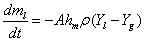(8)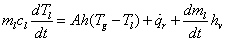(9) where,( ml) is the mass of particle or droplet, (A) is liquid droplet area, (hm) is the coefficient of mass transfer to be discussed below, (ρ) is the density of gas, (cl) is the specific heat of liquid, (h) is the coefficient of heat transfer between the gases and the liquids, ( r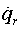) is the radiative rate heating of the particle or droplet, and( hv) is the vaporization latent heat of the liquid. In the equation of mass transfer (7), (Yg) is the mass of vapor fraction of the gas which obtained from the mass conservation equations of gas phase and (Yl) is the equilibrium vapor of liquid mass fraction obtained from the Clausius-Clapeyron equation [12,13]. Heat and mass transfer between gases and liquids are described with analogous empirical correlations. The coefficient of mass transfer, (hm) and (h) the coefficients of heat transfer are described by the empirical relationships .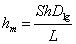(10)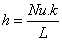(11) Here, Dlg is the mass diffusion coefficient of water vapor in gas mixture;( L) is a scale length equal to the diameter of droplet or particle. (k) is the gas thermal conductivity. The Sherwood number Sh is evaluated from the Ranz and Marshall correlation  as follows: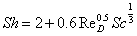(12) (ReD) is the droplet Reynolds number (according to the diameter, D, and the relative air velocity -droplet velocity). The Nusselt number Nu is evaluated with an analogous relationship as Sherwood number Sh,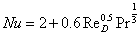(13) Break-Up Model Break up model occurs when the droplet impacts the surface at incoming the Weber number equal or greater than the critical Weber number. The disintegrated number of droplets or particles will increases with the increases in Weber number. This is because of the impact energy and the deformation of the droplet increase with the Weber number [14,15]. The drag force Fd acts as a deforming force. The surface tension on the other hand acts as a restoring force. The contracting force around the perimeter of a droplet, Fσ is given by the surface tension σ multiplied by the length of the circumference: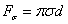(14) The criterion of break-up can be expressed in terms of the relative velocity of droplet and the gas flow, Weber number which is the ratio between the inertia and surface tension forces( can be used to indicate the droplet shape and eventually break up) given by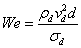(15) Where σd is the water surface tension. The break-up of water mist droplets is modeled by Reitz and Diawaker model . In their model, a two droplets or particles break up regimes are considered. These are bag break-up when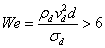(16) And stripping break up when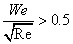(17) Drag Model Simulation In FDS, the equation of motion for a single spherical droplet is determined by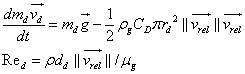(18) Where (md) is the droplet or particle mass,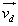is the droplet velocity, ρg is the density droplet of the surrounding gas.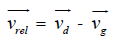(19) Where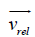the droplet or particle velocity relative to the surrounding gas is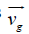is the gas velocity. (Red) is the Reynolds number of droplet or particle . (CD) The coefficient of drag is given by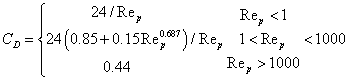(20) According the value of Re ,the CD will be calculated (CD as a function of Re number). A curve representing the approximate expression for drag force coefficient for Renolds Number between [0.5,1] (Figure 2). The arrangement of two droplets is directly in line, the hydrodynamic reduction forces to the second (trailing) sphere because of the wake effect were discussed . They studied in the next analytical formula for the force of hydrodynamic to the second sphere.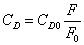(21) Where (CD0) is the drag coefficient of single droplet and (F/F0) is the ratio of hydrodynamic force of trailing droplet or particle to single droplet:(22) Where (Re1) is the Reynolds number of single droplet, (L) is the distance between the droplets and (W) is the non-dimensional velocity in the center of the trailing droplet or particle.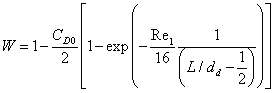(23) The FDS model assumes that the spheres are travelling directly inline with each other. Particle Size Distribution The distribution of droplet sizes in a spray in terms of an idealized droplet size distribution. This could facilitate comparison between various sprays, algebraic manipulation, theoretical analyses and interpolation/extrapolation of data. A variety of model droplet size distributions are in common use, and are either empirical (e.g. Rosin-Rammler, log-normal, root-normal, etc.) or analytical (e.g. Maximum Entropy, and Discrete Probability Function). Analytical approaches are based on conservation of mass, momentum, surface energy, kinetic energy. Detailed descriptions of these distributions are available in [18,19]. A complete plot of the droplet sizes is a better expression than the single values described above. It is possible for two sprays having, for example, the same volume mean diameter, to have widely differing ranges of droplet sizes. The cumulative volume fraction of droplet or particle diameters in FDS follows a distribution that is a combination of lognormal and Rosin-Rammler distributions: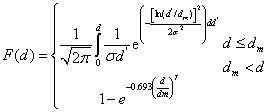(24) By default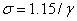Droplets or particles with diameters smaller than dmin are assumed to vaporize instantly. The operating pressure effect on the median droplet size. The determining of experimental droplet size distribution at certain pressure this variation in droplet size is taken into account by scaling the median droplet size as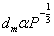The parameters of droplet size distribution were found by least squares fit of the mathematical form of the FDS droplet size spectrum to the experimentally calculated cumulative volume distribution at low pressures Figure 3 . Fitting the FDS cumulative number distribution to the experimentally measured cumulative number distribution was also tested and it was discovered that these two methods resulted in significantly different distribution parameters. Specified Output The following quantities are specified to be recorded in output files from the standard output generated by FDS: a) Droplet size and speed devices measurement (PDPA) are positioned vertically at 70 cm and 100 cm below the nozzle. b) The pressure and droplet flux devices measurement (PDPA) are positioned vertically at 70 cm and 100 cm below the nozzle. c) The drag force of droplet. Results and Discussion In present study, the drag coefficient is calculated numerically for a single droplet moves vertically down .the droplet velocity was investigated from FDS simulation. In Figure 4 a curve representing the approximate expression for drag force coefficient for Reynold’s Number, was used for computing drag force. The droplets will slow down Due to the frictional force; also the maximum velocity it can reach is when the net force is equal zero in equation (18) (Figure 5). The falling rate of droplet as it passes through the plume. As a droplet becomes smaller the drag force created across its surface becomes larger in relation to its mass and hence the gravitational force on it. Hence large droplets will fall quickly to the floor while small droplets will fall at a much slower rate. If the velocity within the plume is higher than falling rate then the droplet will be lifted within the plume and circulated within the compartment (Figure 6). The particle or droplet size distribution parameters are presented by least squares fit of the mathematical form of the FDS droplet size spectrum determined cumulative volume distribution for present study case can be seen in Figure 6. In this section the specifications and characteristics for nozzle B were used with present model. The fire extinction performance of water mist is dependent on the characteristic parameters of velocity distribution, droplets or particle size distribution and flux. Such parameters can be measured using the Phase Doppler Particle Analyzer (PDPA) system. Initial velocity distribution of water mist will affect the performance of the suppression system. The speed of the droplet reduces due to drag and possibly energy lost in droplet collisions (Figure 7). In Figure 8, the slice at center of room or nozzle shows the velocity of droplets decrease. The momentum regime occurs when water mist exits from the nozzle and breaks up into small droplets or particles. The gravitation regime occurs after the droplets begin to reach their respective settling velocities. The smaller droplets are entrained into the spray center, which is formed by the air currents produced by the nozzle. The majority of spray water flux is contained in the spray core. The remainder of the larger droplets has sufficient momentum to reach the outer edges of the spray. Figure 8 also shows the location of the measurement points 70 and 100 cm below the nozzle in the transition regime. Although measurements were taken at all points, data was analyzed only up to a radial distance of 15 cm, as the images from the outer measurement points contained very little droplets. A droplet size distribution can be presented in terms of surface area, volume, or number (i.e. count) as mentioned above. When fitting a dataset to a distribution, it is therefore important to choose a form appropriate to the source of the data, and the intended application of the model distribution. In the next Figures presents the profiles for average droplets diameter, average mean velocity, and average mist flux. The average values at each distance are calculated over the four measuring points at that distance (except for the point at the spray axis). In Figure 9, the maximum velocity in the center of the spray the velocity profile becomes broader further away from the nozzle. Maximum velocity reduces from away the center of spray. The water concentration in the spray at the center reaches a maximum .the water concentration levels out and water becomes relatively evenly distributed over the area .as can be shown in slice Figure 10. Droplets size further vary within the spray field as the sample point moves from the central axis of the nozzle out to the perimeter of the spray pattern, as well as with distance from nozzle orifice. The predicted diameter profile can be seen in Figure 11. A flat mean diameter profile is predicted for nozzle. In short beside or near of the nozzle, the entrained air will tend to pull droplets or particles towards the centre of the spray. Smaller droplets or particles have shorter times response and thus are entrained more easily. These processes should result in a diameter profile that shows more small droplets or particles in the centre of the spray and larger droplets from away the centre or (on the spray boundaries). In Figure 12, the water mist flux is high in the center of spray and reduces whenever away from the center. The velocity in the canter of the jet is higher than further out, leading to larger droplets velocities and higher mist flux. The increase in droplets diameter is caused by entrainment. In Figure 13, it shows that a parent (initial) droplet or particle is breaking up into many child water mist droplets. Also the child droplets diameter decreases as a function of the number of fragments formed can be seen in Figure 14. Figures 14-16 show the relation between numbers of droplets with radial distance for two positions of measurement device at 70, 100 cm below the nozzle. It can be seen the number of droplets is maximum in the center of spray and otherwise. Conclusion A FDS program is used to simulate the fire compartment and show output results of the droplet velocity and diameter of droplet for several models. After running many computer simulations, the following conclusions are estimated: • The droplet diameter, droplet speed, water mist flux, number of droplet and droplets distribution were simulated in case of normal room. • The flux of water mist is high at the center of spray and reduces whenever away from the center. • The droplet speed is highest in the center of the spray .the velocity profile becomes broader further away from the nozzle. Maximum velocity reduces from away the center of spray. References Hostikka S, Vaari J, Sikanen T, Paajanen A (2011) Proceedings: Fire and Evacuation Modeling Technical Conference, Baltimore, Maryland, August 15-16, 2011 Wigus R, Aune P, Drangshot G, Stensaas JP (1993) Fine Water Spray System Extinguishing Tests in Medium and Full Scale Turbine Hood. P Int Water Mist Conference, Swedish Testing Institute, Boras, Sweden, Nov. 5-7. Ndubizu CC, Ananth R,Tatem PA (2000) The effects of droplet size and injection orientation on water mist suppression of low and high boiling point liquid pool fires. Combust Sci Technol 157: 63–86. Simpson T,Smith DP (1993) A Fully integrated water mist fire suppression system or Telecommunications and other electronics cabinets. Fire and Safety International, Colnbrook, UK. Aderson P,Arvidson M, Holinstedt G (1996) Small scale experiments and theoretical aspects of flame extinguishment with water mist. Lunds tekniskahogskola, Lund University. Evans D,Pfenning D (1985) Water sprays suppress gas-well blowout fires. Oil and Gas J. Hua H, Kumar K, Khoo BCJ (2002) A numerical study of the interaction of water spray with a fire plume. Fire Safety J 37: 631–657. http://fdssmv.googlecode.com/svn/trunk/fds/trunk/manuals/all_PDF_files/FDS_5_technical_reference_guide.PDF Anderson DA, Tannehill JC, Pletcher RH (1984) Computational fluid mechanics and heat transfer.hemisphere publishing corporation, Philadelphia, Pennsylvania,11,15. Hamdan S, Klaus-Jürgen K, Reinhard G (2009) Interaction of water mist with fire plume by using FDS. Jahresbericht 2006/2007/2008, IdF Sachsen-Anhalt, Heyrothsberge. Incropera FP, De Witt DP (1996) Fundamentals of heat and mass transfer. John Wiley and Sons, (4thedn). New York, McGrattan KB, Hostikka S, Floyd JE, Baum HR, Rehm RG (2007) Fire dynamics simulator (Version 5)”, technical reference guide. NIST Special Publication 10185, National Institute of Standards and Technology, Gaithersburg, Maryland. Ranz WE, Marshall WR (1952) Evaporation from droplet: part II. Chemical Engineering Progress, 48: 173–180. Hatta N (1997) Experimental study of deformation mechanism of a water droplet impinging on hot metallic surfaces above Leiden frost Temperature. Transactions of the ASME 119. Hatta N(1995) Collision dynamic of water droplet impinging on a rigid surface above Leiden frost Temperature. ISIJ int 35. Diwakar R, Reitz RD (1986) Effect of droplets breakup on fuel sprays. SAE Report 860469. Ramírez-MJ, Soria A, Salinas-RE (2007) Hydrodynamic force on interactive spherical particles due to the wake effect. Int J Multiphase Flow 33: 802–807. Crowe C, Sommerfeld M, Tsuji Y (1988) Multiphase flows with droplets and particles. CRC Press. Babinsky E, Sojka, PE (2002) Modelling droplets size distributions. Progress in Energy and Combustion Science.

#### Figures at a glance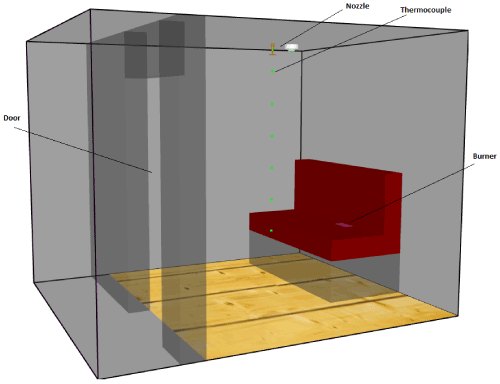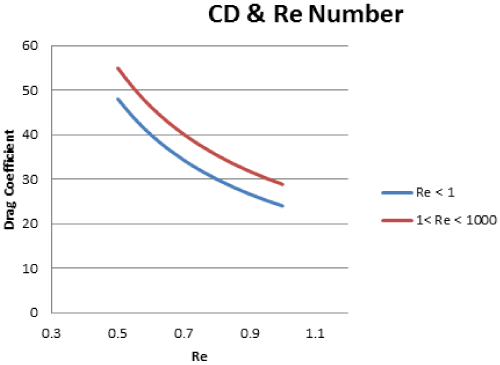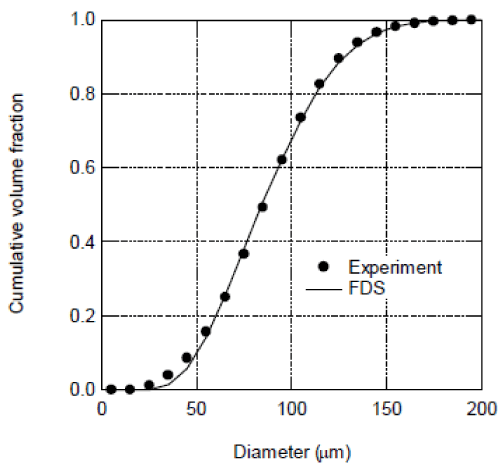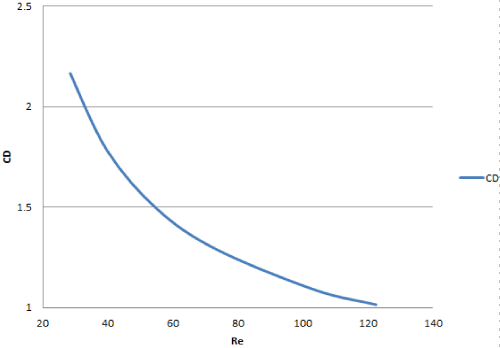Figure 1 Figure 2 Figure 3 Figure 4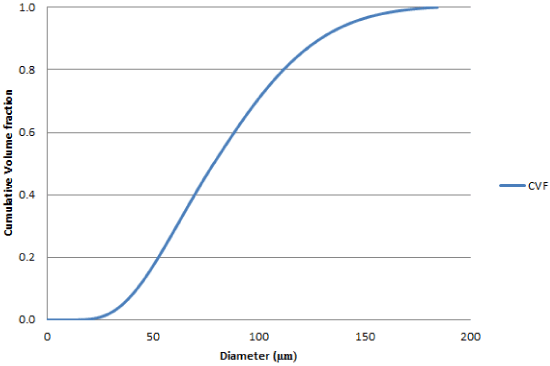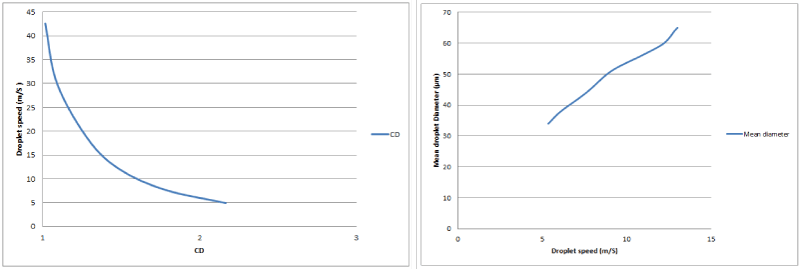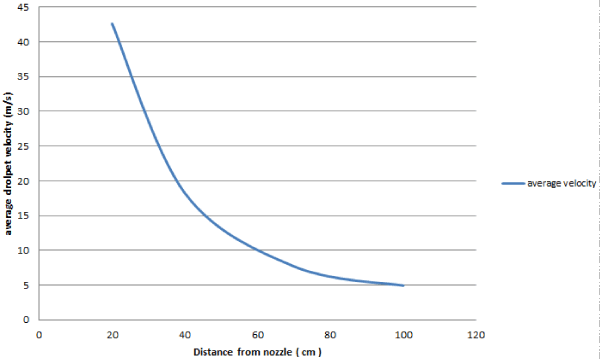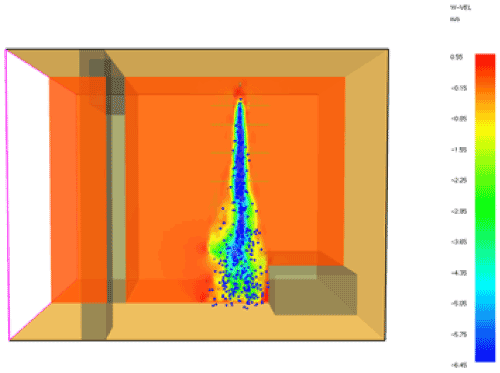Figure 5 Figure 6 Figure 7 Figure 8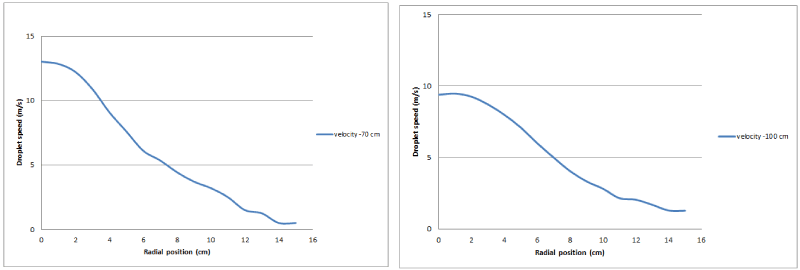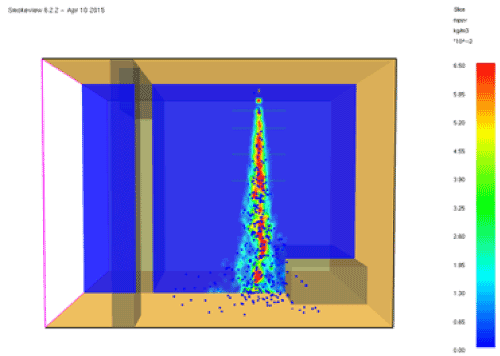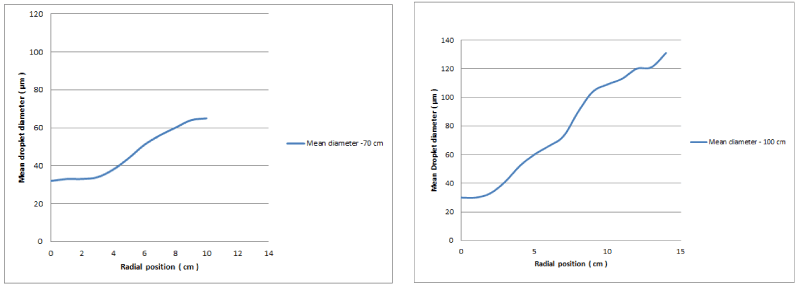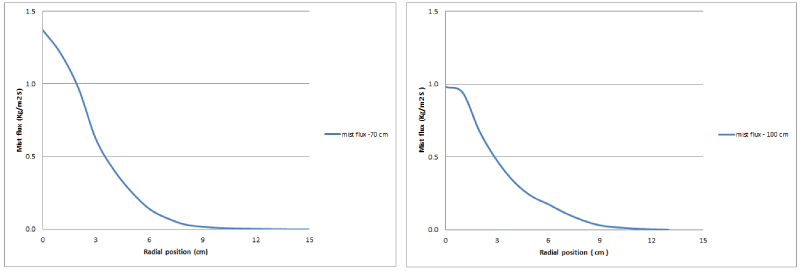Figure 9 Figure 10 Figure 11 Figure 12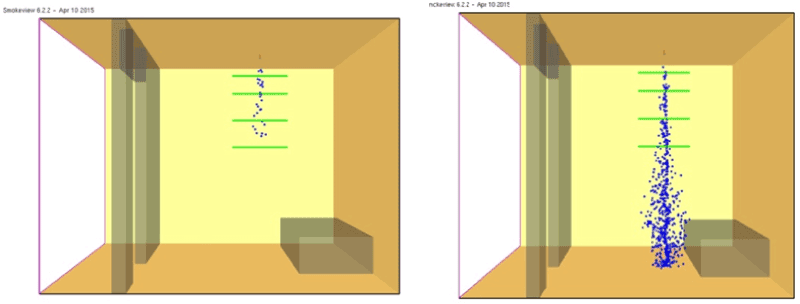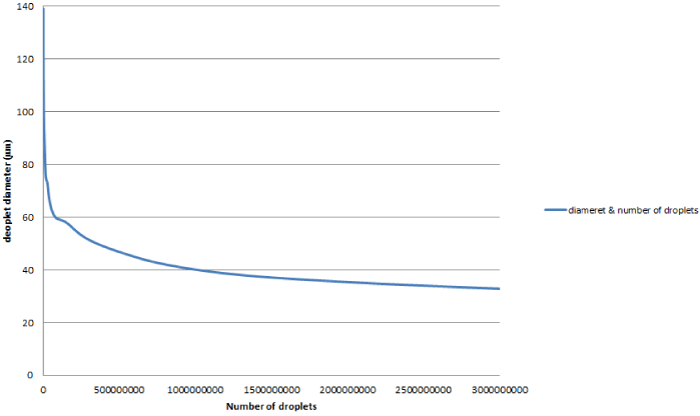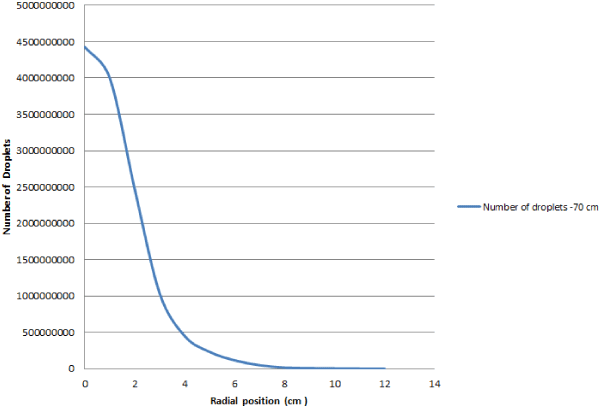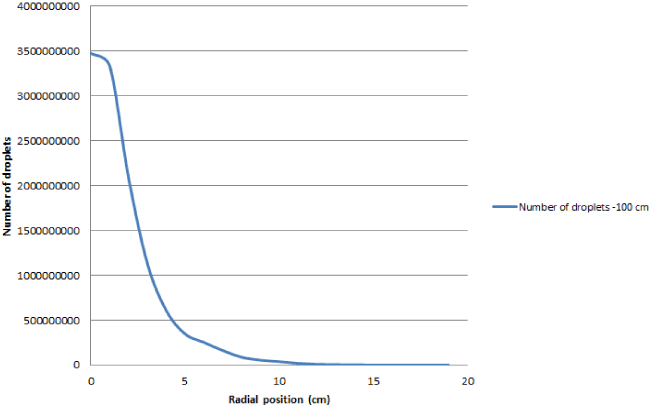Figure 13 Figure 14 Figure 15 Figure 16
Select your language of interest to view the total content in your interested language

### Article Usage

• Total views: 9087
• [From(publication date):
June-2016 - Jan 22, 2020]
• Breakdown by view type
• HTML page views : 8916

## Review summary

1. Mutawassit
Posted on Oct 12 2016 at 2:32 pm
This paper is focusing on investigating the water mist droplet using fire dynamic simulator. The whole paper gave the droplet models and specified the case for investigation. And the results show that the flux of water mist reduces away from the center, as well as the velocity profile becomes broader. The statements in the paper are clear and reasonable.
2. Lee Scott
Posted on Sep 23 2016 at 6:30 pm
It is good report and this paper is explains about the fire extinguishers using water mist. They explained clearly about various drop let size of the water mist and nozzle flow which was studied by using FD. They also explained gas concentration affecting fire extinguishing time. Using water as fire extinguisher is good as it is cost effective.Can't read the image? click here to refresh